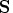Previous Page | Next Page

 Language Reference

## NROW Function

finds the number of rows of a matrix

NROW( matrix)

where matrix is a numeric or character matrix.

The NROW function returns a single numeric value that is the number of rows in matrix. If the matrix has not been given a value, the NROW function returns a value of 0.

For example, to let J contain the number of rows of the matrix, use the following statement:

```
j=nrow(s);
```Previous Page | Next Page | Top of Page